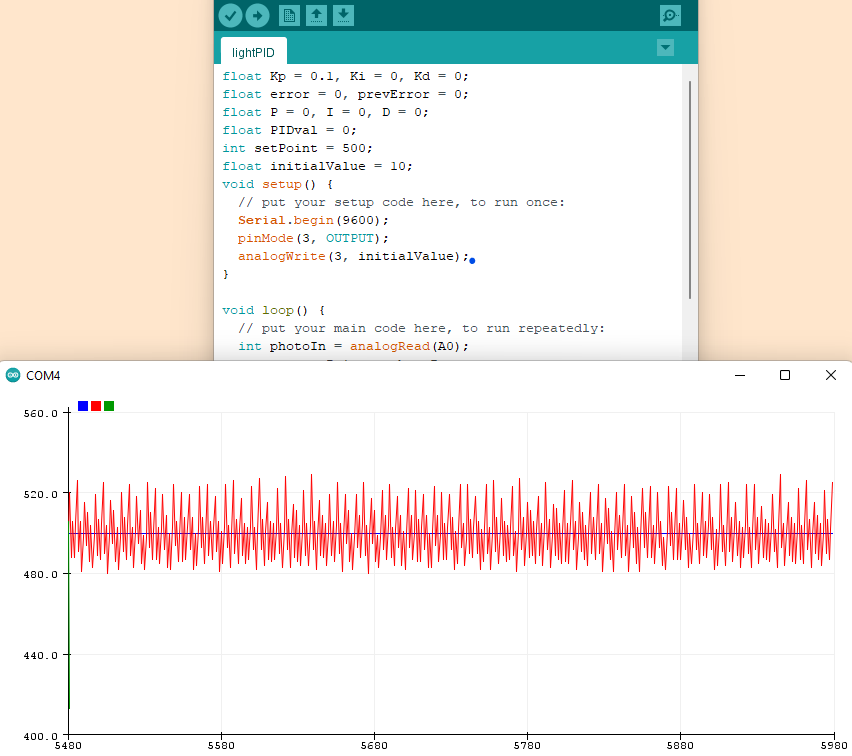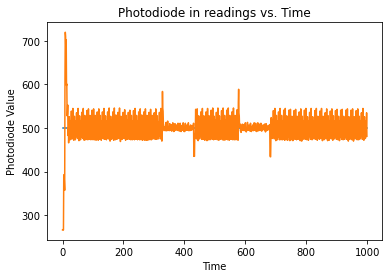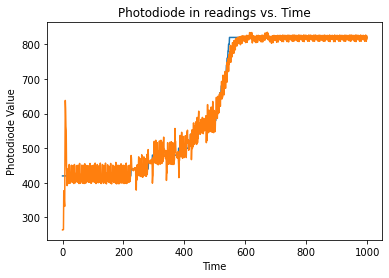CE 351 Spring 2023
Lab 6 Photo PID
Name:
Photo PID

Introduction: Using a PID loop to stabilize LED brightness
Materials:
Photocell, LED, arduino

Methods: The background light was measured with the LED off and the ambient light was 337, the LED was then turned on to read 641 so I set my setpoint to 500.Figure 1. Light oscilating around the setpoint, 500.
A python script was then written to interface with the serial port that the arduino was reading into. Matplotlib was used to make a real time plot over the course of 2 seconds and can be seen below in Figure 3. Spikes can be seen where the button was pressed turning on the white led followed by a fall when the button was released. I think the noise reducess while the white light is on because the white light provides a more stable reading over the red LED.Figure 2. Matplotlib plot of serial read in from photodiode.

Finally, the equilibrium point was increased by wiring the button to digital 4 and writing a brief script to check if the button was pressed before increasing the setpoint. The python graph of this can be seen below in Figure 3. The blue line is the setpoint and the orange line is the read in value of the photodiode.Figure 3.
Matplotlib plot of serial read in from photodiode with increasing setpoint.

This lab was fairly straightforward as the PID values were not difficult and the wobble in value was not crucial like a balancing robot.